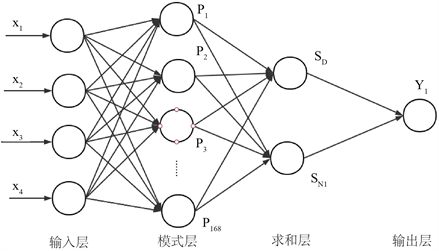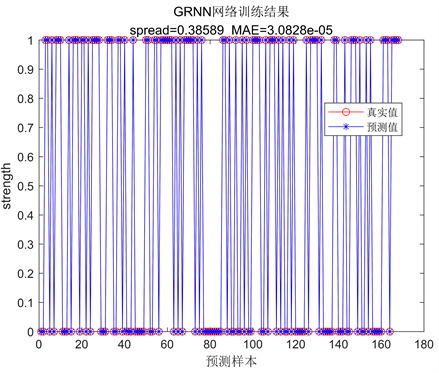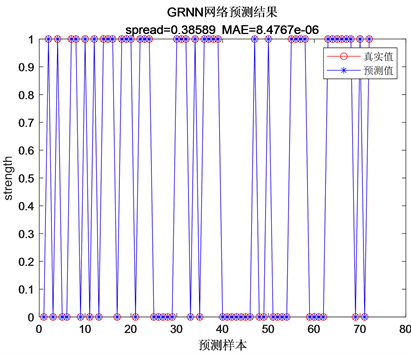﻿ 基于改进GRNN的电网雷击跳闸预测

# 基于改进GRNN的电网雷击跳闸预测Predicting Lightning Outages of Transmission Lines Using Modified Generalized Regression Neural Network

Abstract: The security, reliability and stability of power system are of great significance. Tripping caused by lightning strikes on transmission lines is a common form of hazard. Therefore, it is of great signifi-cance to effectively prevent and predict lightning strikes. Aiming at the problem of optimal selec-tion of hyperparameters in generalized regression neural networks (GRNN), this paper proposes an optimal hyperparametric method based on harmony search (HS) algorithm. Then, the improved method is applied to establish the prediction model of lightning strikes. Fault detection rate (FDR), false alarm rate (FAR), total prediction accuracy (PA) and mean absolute error (MAE) are adopted to evaluate the prediction performance. The test results indicate that the prediction model of light-ning strikes based on improved GRNN can accurately predict lightning outages with an outstanding performance.

1. 引言

2. 基于改进GRNN的雷击跳闸预测模型

2.1. 雷击跳闸过程分析

$P=\eta \left(g{P}_{1}+{P}_{a}{P}_{2}\right)$ (1)

2.2. 基于改进GRNN的雷击跳闸预测步骤

2.3. 预测性能的评价指标

$\text{FDR}=\frac{FN}{FP+FN}×100\text{%}$ (2)

$\text{FAR}=\frac{TN}{TP+TN}×100\text{%}$ (3)

$\text{PA}=\frac{FN+TP}{FP+FN+TP+TN}×100\text{%}$ (4)

$\text{MAE}\left(y,\stackrel{^}{y}\right)=\frac{1}{n}\underset{i=1}{\overset{n}{\sum }}|y-\stackrel{^}{y}|$ (5)

3. 仿真实验

3.1. 模型建立Table 1. Statistic of lighting trip information (Part)

3.2. 基于HS-GRNN的雷击跳闸预测模型训练Figure 1. GRNN structure applied to predicting lighting outagesFigure 2. HS-GRNN predicting model training results

3.3. 基于HS-GRNN的雷击跳闸预测模型测试Figure 3. HS-GRNN predicting model testing resultsTable 2. The statistics of predicting results by HS-GRNN

3.4. 基于HS-GRNN的雷击跳闸预测结果与其他模型预测结果的对比Table 3. Predicting results of different NN

4. 结束语

 Hu, Y., et al. (2014) Analysis of Influential Factors on Operation Safety of Transmission Line and Countermeasures. High Voltage Engineering, 40, 3491-3499.

 陈继东, 吴伯华. 线路型500 kV避雷器保护范围的研究[J]. 电磁避雷器, 2002(5): 33-38.

 IEEE Working Group on Estimating Lightning Performance of Transmission Lines (1985) A Simplified Method for Estimating Lightning Performance of Transmission Lines. IEEE Transactions on Power Apparatus and Systems, PAS-104, 918-932.
https://doi.org/10.1109/TPAS.1985.319093

 杨清, 熊小伏, 王建, 翁世杰. 一种输电线路雷击概率预测新方法及其在书店系统短期可靠性评估中的应用[J].智能电网, 2014, 4(2): 37-46.

 Xie, Y.Y., Li, C.J., Lv, Y.J. and Yu, C. (2019) Predicting Lightning Outages of Transmission Lines Using Generalized Regression Neural Network. Applied Soft Computing Journal, 78, 438-446.
https://doi.org/10.1016/j.asoc.2018.09.042

 Yao, C., et al. (2014) Study of Magnetic Fields from Different Types of Lightning Faults on a Multi-Tower System. IEEE Transactions on Dielectrics and Electrical Insulation, 21, 1866-1874.
https://doi.org/10.1109/TDEI.2014.004324

 Hunt, H.G.P., et al. (2020) Can We Model the Statistical Distribution of Lightning Location System Errors Better? Electric Power Systems Research, 178, 1-10.
https://doi.org/10.1016/j.epsr.2019.106042

 Morales, J.A., Anane, Z. and Cabral, R.J. (2018) Automatic Lightning Stroke Location on Transmission Lines Using Data Mining and Synchronized Initial Travelling. Electric Power Systems Research, 163, 547-558.
https://doi.org/10.1016/j.epsr.2018.01.025

 Geem, Z.W., et al. (2001) A New Heuristic Optimization Algorithm: Harmony Search. Simulation, 76, 60-68.
https://doi.org/10.1177/003754970107600201

Top